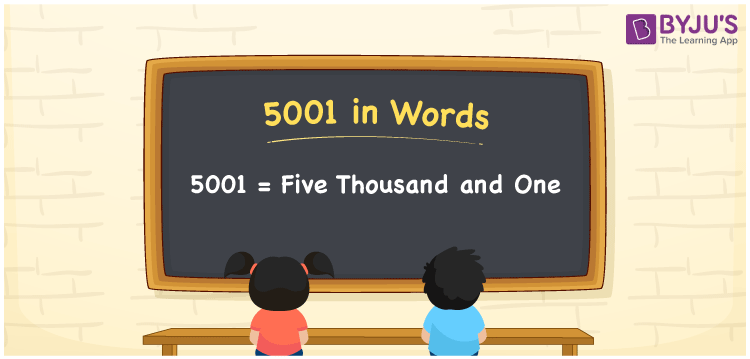# 5001 in Words

5001 in words is Five thousand and one. As we know, while converting numbers into words, the place value system is very important. The number 5001 has four digits and identifying the place values of each digit helps to write the number name. Here, we have provided the complete explanation of writing the number 5001 in words.

 5001 in Words: Five Thousand and One. Five Thousand and One in Numerical Form: 5001.

## 5001 in English Words## How to Write 5001 in Words?

The number 5001 has four digits and its place value table up to four digits is given below:

 Thousands Hundreds Tens Ones 5 0 0 1

The expanded form of 5001 is as follows:

= 5 × Thousand + 0 × Hundred + 0 × Ten + 1 × One

= 5 × 1000 + 0 × 100 + 0 × 10 + 1 × 1

= 5000 + 1

= 5001

= Five thousand and one

Hence, 5001 in words is five thousand and one.

5001 in words – Five thousand and one

Is 5001 an odd number? – Yes

Is 5001 an even number? – No

Is 5001 a perfect square number? – No

Is 5001 a perfect cube number? – No

Is 5001 a prime number? – No

Is 5001 a composite number? – Yes

## Frequently Asked Questions on 5001 in Words

Q1

### How to write 5001 in words?

5001 in words is five thousand and one.

Q2

### Simplify 5000+1, and express it in words.

Simplifying 5000+1, we get 5001. Hence, 5001 in words is five thousand and one.

Q3

### Is 5001 an odd number?

Yes, 5001 is an odd number.